Filter By

## All Questions

#### A is a point at a distance 13 cm from the centre O of a circle of radius 5 cm.AP and AQ are the tangents to the circle at P and Q. If a tangent BC is drawn at a point R lying on the minor arc PQ to intersect AP at B and AQ at C, find the perimeter of the ABC.

Solution
Let us make figure according to question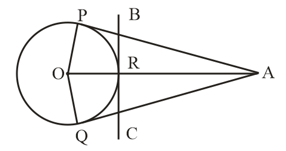Given : OA = 13 cm, Radius = 5 cm
Here AP = AQ            (tangent from same point)
OP  PA, OQ QA  (  AP, AQ are tangents)
In OPA using Pythagoras theorem

........(i)
Perimeter of ABC = AB + BC + CA
= AB + BR + RC + CA
= AB + BD + CQ + CA
[ BP and BR are tangents from point B and CP and CQ are tangents from point C]
= AP + AQ      [  AP = AB + BP, AQ = AC + CQ]
= AP + AP      [  AP = AQ]
= 2AP
= 2 × 12           [using (i)]
= 24 cm

#### If an isosceles triangle ABC, in which AB = AC = 6 cm, is inscribed in a circle of radius 9 cm, find the area of the triangle.

Solution
According to question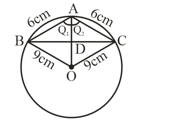In and
AB = AC        [Given]
AO = AO        [Common side]
[By SSS congruence Criterion]
[CPCT]
In and
AB = AC        [given]

[By SAS congruence Criterion]
…..(i)   [CPCT]
…..(ii)
From (i) and (ii)

OA is a perpendicular which bisects chord BC
Let AD = x, then OD = 9 – x

…..(iii)
In ODC using Pythagoras theorem

…..(iv)
From (iii) and (iv)

i.e., AD = 2 cm, OD = 9 – 2 = 7 cm
Put value of x in (iii)

Areao of

## Crack CUET with india's "Best Teachers"

• HD Video Lectures
• Unlimited Mock Tests
• Faculty Support#### The tangent at a point C of a circle and a diameter AB when extended intersect at P. If PCA = , find CBA [see Figure].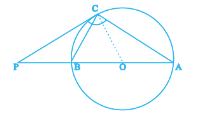Solution
Given :
Here
[  PC is tangent]

[  Sides opposite to equal angles are equal]
We know that PC is tangent and angles in alternate segment are equal.
Hence
In
[Interior angles sum of triangle is 180°]
[using (i)]
…..(ii)
Here

( using (ii))
…..(iii)
In
[using (iii)]

Hence

#### In Figure. O is the centre of a circle of radius 5 cm, T is a point such that OT = 13 cm and OT intersects the circle at E. If AB is the tangent to the circle at E, find the length of AB.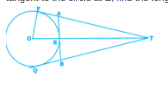Solution
Given: Radius = 5 cm, OT = 13 cm
[  PT is tangent]
Using Pythagoras theorem OPT

PT and QT are tangents from same point
PT = QT = 12 cm
AT = PT – PA
AT = 12 – PA        ..…(i)
Similarly BT = 12 – QB          ..…(ii)
Since PA, PF and BF, BQ are tangents from point A and B respectively.
Hence, PA = AE              ..…(iii)
BQ = BE     ..…(iv)
AB is tangent at point E
Hence OE  AB

ET = OT – OF
ET = 13 – 5
ET = 8 cm
In AET, using Pythagoras theorem

[using (i) and (ii)]

Similiraly

## Crack NEET with "AI Coach"

• HD Video Lectures
• Unlimited Mock Tests
• Faculty Support#### In Figure, the common tangent, AB and CD to two circles with centres O and O' intersect at E. Prove that the points O, E, O' are collinear.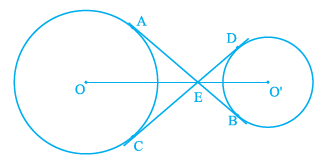Solution
Construction : Join AO and OS
and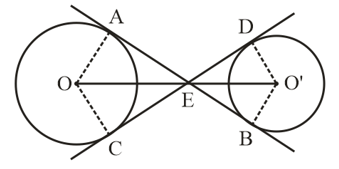In and
[Common side]
ED = EB           [Tangent drawn from an external point to the line circle  are equal to length]
[By SSS congruence criterion]

i.e.,       is bisector of
Similarly OE is bisector of

[  AB is a straight line]
[From equation (ii)]

Similarly
From equation (ii)
Dividing both side by 2

Similarly
Dividing both side by 2

Now

[from equation (iii) and (iv)]

So,OEO’ is straight line
O, E and  are collinear.
Hence Proved

#### Prove that the tangent drawn at the mid-point of an arc of a circle is parallelto the chord joining the end points of the arc.

Solution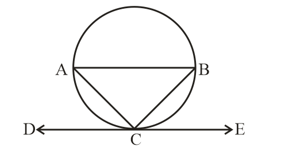Let mid-point of arc is C and DCE be the tangent to the circle.
Construction: Join AB, AC and BC.
Proof: In ABC
AC = BC

[  sides opposite to equal angles are equal]
Here DCF is a tangent line
[  angle in alternate segments are equal]
…..(ii)         [From equation (i)]
But Here  and  are alternate angels.
equation (ii) holds only when AB||DCE.

Hence the tangent drawn at the mid-point of an arc of a circle is parallel to the chord joining the end points of the arc.

Hence Proved.

## Crack JEE Main with "AI Coach"

• HD Video Lectures
• Unlimited Mock Tests
• Faculty Support#### AB is a diameter and AC is a chord of a circle with centre O such that BAC = . The tangent at C intersects extended AB at a point D. Prove that BC = BD.

Solution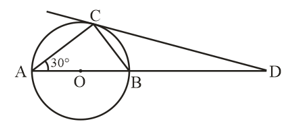Given AB is a diameter and AC is a chord of circle with center O.

To Prove : BC = BD
Construction : Join B and C
Proof :[Angle is alternate segment]

[Angle in semi circle formed is 90°]
In ABC
[Sum of interior angles of a triangle is 180°]

Also ,  [Linear pair]

In

From equation (i) and (ii)

[  Sides opposite to equal angles are equal]
Hence Proved

#### In Figure, tangents PQ and PR are drawn to a circle such that RPQ = . A chord RS is drawn parallel to the tangent PQ. Find the RQS.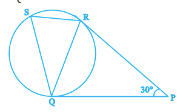Solution
In the given figure PQ and PR are two tangents drawn from an external point P.
PQ = PR      [ lengths of tangents drawn from on external point to a circle are equal]
[  angels opposite to equal sides are equal]
In PQR

[  sum of angels of a triangle is 180°]

SR||OP (Given)

[Alternate interior angles]

Also       [Alternate segment angles]
In QRS

## Crack CUET with india's "Best Teachers"

• HD Video Lectures
• Unlimited Mock Tests
• Faculty Support#### In a right triangle ABC in which B = , a circle is drawn with AB as diameter intersecting the hypotenuse AC and P. Prove that the tangent to the circle at P bisects BC.

Solution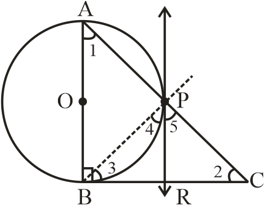Let O be the center of the given circle.
Suppose P meet BC at point R
Construction: Join point P and B
To Prove: BR = RC
Proof:   [Given]
In ABC
[  Sum of interior angle of a triangle is 180°]

Also              [  tangent and chord made equal angles in alternate segment]

[  angle in semi circle formed is 90°]

Equal equation (i) and (ii) we get

[Side opposite to equal angles are equal]
Also,    PR = BR         …..(iv)   [  tangents drawn to a circle from external point are equal]

From equation (iii) and (iv)
BR = RC

Hence Proved

#### Two circles with centres O and O' of radii 3 cm and 4 cm, respectively intersect at two points P and Q such that OP and O'P are tangents to the two circles. Find the length of the common chord PQ.

4.8 cm
Solution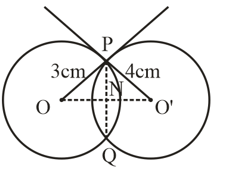Given : Radii of two circles are OP = 3 cm and  and intersection point of two circles are P and Q.  Here two tangents drawn at point P are OP and P

Also apply Pythagoras theorem in  we get

Equate equation (i) and (ii) we get

Put x = 1.8 in equation (i) we get